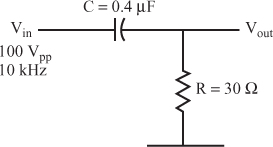# Self-Test

For questions 1–3, calculate the following parameters for the circuit shown in each question.

A. XC
B. Z
C. Vout
D. I
E. tan θ and θ
1. Use the circuit shown in Figure 6.53.

Figure 6.53A. _____
B. _____
C. _____
D. _____
E. _____
2. Use the circuit shown in Figure 6.54.

Figure 6.54A. _____
B. _____
C. _____
D. _____
E. _____
3. Use the circuit shown in Figure 6.55.

Figure 6.55A. _____
B. _____
C. _____
D. _____
E. _____

For questions 4–6, calculate the following parameters for the circuit shown in each question.

A. XC
B. AC Vout
C. DC Vout
4. Use the circuit shown in Figure 6.56.

Figure 6.56A. _____
B. _____
C. _____

5. Use the circuit shown in Figure 6.57.

Figure 6.57A. _____
B. _____
C. _____
6. Use the circuit shown in Figure 6.58 ...

Get Complete Electronics Self-Teaching Guide with Projects now with the O’Reilly learning platform.

O’Reilly members experience books, live events, courses curated by job role, and more from O’Reilly and nearly 200 top publishers.